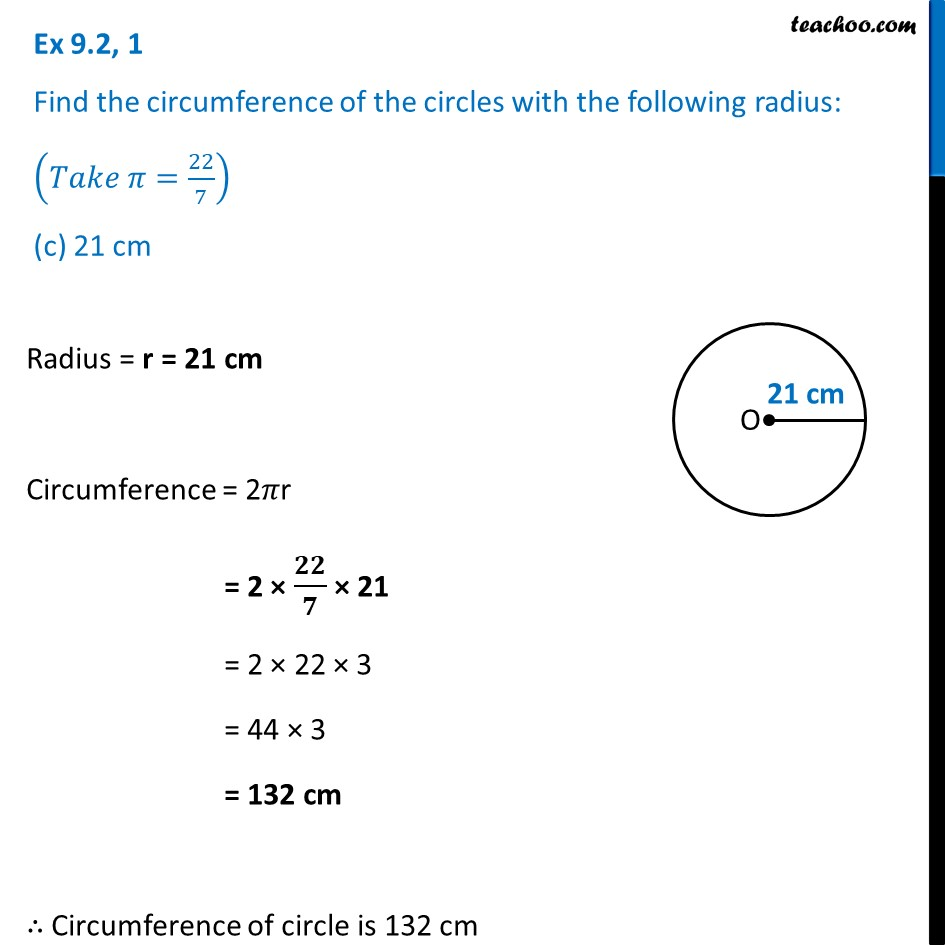Ex 9.2

Chapter 9 Class 7 Perimeter and Area
Serial order wiseLearn in your speed, with individual attention - Teachoo Maths 1-on-1 Class

### Transcript

Ex 9.2, 1 Find the circumference of the circles with the following radius: (𝑇𝑎𝑘𝑒 𝜋=22/7) (c) 21 cmRadius = r = 21 cm Circumference = 2𝜋r = 2 × 𝟐𝟐/𝟕 × 21 = 2 × 22 × 3 = 44 × 3 = 132 cm ∴ Circumference of circle is 132 cm